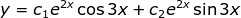Friday, March 20, 2015

Eliminating Arbitrary Constant, 20

Category: Differential Equations

"Published in Newark, California, USA"

Eliminate the arbitrary constant for

Solution:

Consider the given equation above

Take the derivative on both sides of the equation with respect to x, we have

Since there are two constants in the given equation, then we need to take the derivative again with respect to x for the above equation as follows

Multiply the first derivative equation by -4 and then add it to the second derivative equation, we have

-----------------------------------------------------------------------------

but